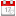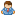jquery中判断某一值是否属于数组2013-04-22 17:23:01阿炯

Javascript/Jquery Convert string to array-将字符串转换为数组
var traingIds=('a','b','d');
var trainindIdArray = traingIds.split(',');

var type = typeof(traingIds);

---------------

1、使用'jquery'中的'inArray'函数
jQuery.inArray()
jQuery.inArray( value, array [, fromIndex ] )
Description: Search for a specified value within an array and return its index (or -1 if not found).

The \$.inArray() method is similar to JavaScript's native .indexOf() method in that it returns -1 when it doesn't find a match. If the first element within the array matches value, \$.inArray() returns 0.

Because JavaScript treats 0 as loosely equal to false (i.e. 0 == false, but 0 !== false), if we're checking for the presence of value within array, we need to check if it's not equal to (or greater than) -1.

2、使用'grep'函数

jQuery.grep()
jQuery.grep( array, function(elementOfArray, indexInArray) [, invert ] )
Description: Finds the elements of an array which satisfy a filter function. The original array is not affected.

The \$.grep() method removes items from an array as necessary so that all remaining items pass a provided test. The test is a function that is passed an array item and the index of the item within the array. Only if the test returns true will the item be in the result array.

The filter function will be passed two arguments: the current array item and its index. The filter function must return 'true' to include the item in the result array.
-------
Filter an array of numbers to include only numbers bigger then zero.
\$.grep( [0,1,2], function(n,i){
return n > 0;
});

[1, 2]
-------
Filter an array of numbers to include numbers that are not bigger than zero.
arr = jQuery.grep(arr, function(n, i){
return (n != 5 && i > 4);
},true);
\$("p").text(arr.join(", "));
-------
This solution uses the \$.grep() method to filter the months array so that it only includes entries that begin with the capital letter J. The \$.grep method returns the filtered array.
(function(\$) {
var months = [  'January', 'February', 'March', 'April', 'May','June', 'July', 'August', 'September', 'October','November', 'December'];
months = \$.grep(months, function(value, i) {
return ( value.indexOf('J') == 0 );
});
\$(' #months').html( '<li>' + months.join('</li><li>') + '</li>' );
});
})(jQuery);

The callback method defined by the developer takes two arguments and is expected to return a Boolean value of true to keep an element or false to have it removed. The first argument specified is the value of the array element (in this case, the month), and the second argument passed in is the incremental value of the number of times the \$.grep() method has looped.

So, for example, if you want to remove every other month, you could test whether ( i % 2 ) == 0, which returns the remainder of i / 2. (The % is the modulus operator, which returns the remainder of a division operation. So, when i = 4, i divided by 2 has a remainder of 0.)
(function(\$) {
var months = [  'January', 'February', 'March', 'April', 'May','June', 'July', 'August', 'September', 'October','November', 'December'];
months = \$.grep(months, function(value, i) {
return ( i % 2 ) == 0;
});
\$(' #months').html( '<li>' + months.join('</li><li>') + '</li>' );
});
})(jQuery);

var keya=authList.split(',');
//get the items by ajax
\$.ajax({
url: 'items.psp',
//data: "{}",
dataType: "json",
type: "get",
contentType: "application/json; charset=utf-8",
success: function(data){
var json = eval(data);
for (var ind in json){
if(\$.inArray(ind,keya) == -1){
}else{
\$('#items').append(\$("<option value='" + ind + "'>" + json[ind].title + "</option>"));}
}
},
error: function(){